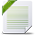# Power BI For Data Science

View Only

## Dynamic (self)reporting with R and PowerBI

• #### 1.  Dynamic (self)reporting with R and PowerBI

Posted Mar 31, 2019 01:24 PM
Dear All,
I would like to create a dynamic scatterplot in which user can choose x, y axis, color coding and maibe more.
Both for aggregated and non aggregated measure (eventually in 2 different sheets).

This because I created it into Tableau using parameters and I would like to recreate same in PowerBI (if technically possible).

My attempt is the followingThe odd thing for me is that to R value are passed non-aggregate even if into the value selection formula is returning measure which is in fact aggregated (even top right mark cards created for debugging show the same), below code chunks:

ValueSelection =
VAR Selection =
SELECTEDVALUE(SlicerTable[Slicer];BLANK())
RETURN
SWITCH(TRUE();
Selection = "Price"; car90[Price_Avg];
Selection = "Disp" ; car90[Disp_Avg];
Selection = "HP"; car90[HP_Avg])

--------------------------------------------------------------------------------------------

Formula created for aggregation

Disp_Avg = AVERAGE(car90[Disp])
HP_Avg = AVERAGE(car90[HP])
Price_Avg = AveragE(car90[Price])
--------------------------------------------------------------------------------------------

My R Script

library(ggplot2)
ggplot(data= dataset) +
geom_point(mapping = aes(x = ValueSelection, y = ValueSelection2 , colour = ValueSelection2)) +
theme(axis.text.x = element_text(angle = 90, hjust = 1))

Is there a more effective to pass R parameters?
Note: I'm doing this in R because I've not seen something similar achieved with power Bi alone.

Thanks to everyone which would like to contribute.
Regards,
Luca

------------------------------
Luca Montanari
------------------------------

Attachment(s)mtcars.pbix   133 KB 1 version